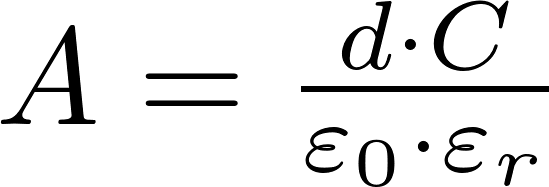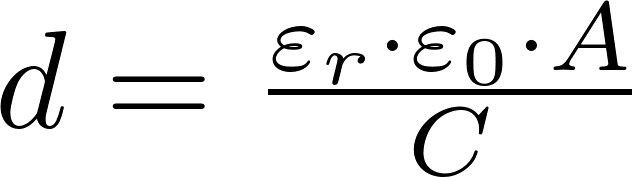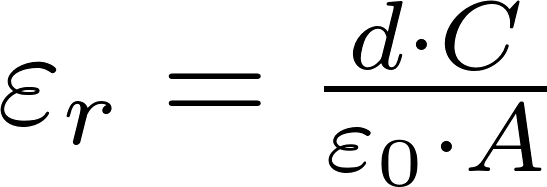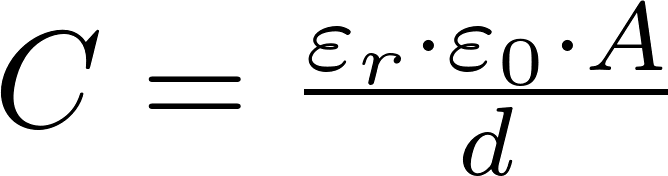# Plate capacitor

The plate capacitor is a type of capacitor consisting of two plates with a dielectric medium in between them. It is one of the simplest type of capacitor.

### FormulasA is the symbol for area and is measured in square meters (m 2 ).
d is the symbol for distance and is measured in meter (m).
ϵ r is the symbol for relative permittivity.
C is the symbol for capacitance and is measured in farad (F).

### Calculator

Enter three values to calculate the remaining one.